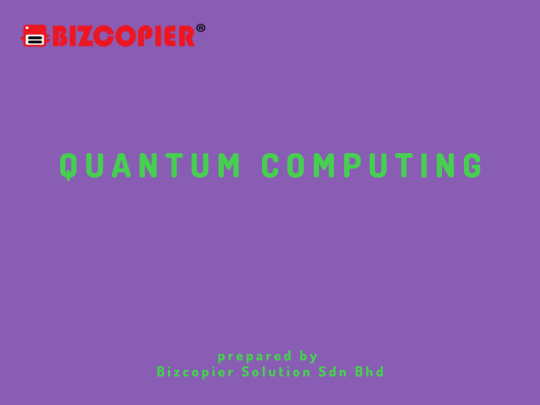# Quantum Computing

## Quantum ComputingQuantum computing is an advanced computational paradigm that leverages the principles of quantum mechanics to perform complex calculations at speeds and scales that would be practically impossible for classical computers. Unlike classical computers, which use bits as the basic unit of information (representing either 0 or 1), quantum computers use quantum bits or qubits, which can exist in multiple states simultaneously due to a phenomenon called superposition.

Key Concepts in Quantum Computing:

1. Superposition: Qubits can exist in a superposition of states, meaning they can represent both 0 and 1 at the same time. This property allows quantum computers to process a vast amount of information in parallel, leading to potential speedups in certain calculations.

2. Entanglement: Entanglement is a phenomenon where qubits become interconnected in such a way that the state of one qubit is dependent on the state of another, even if they are physically separated. This property enables enhanced correlation and coordination between qubits.

3. Quantum Gates: Quantum gates are analogous to classical logic gates but operate on qubits. They manipulate qubits’ quantum states, enabling the execution of quantum algorithms.

4. Quantum Algorithms: Quantum algorithms are designed to take advantage of the unique properties of qubits to solve specific problems more efficiently than classical algorithms. For example, Shor’s algorithm can factor large numbers exponentially faster than the best-known classical algorithms, which has implications for cryptography.

Potential Applications:

Quantum computing has the potential to revolutionize various fields:

1. Cryptography: Quantum computers could potentially break many of the encryption methods used to secure sensitive information, prompting the development of quantum-resistant encryption techniques.

2. Optimization: Quantum computers could be used to solve complex optimization problems, such as optimizing supply chains or financial portfolios, more efficiently than classical computers.

3. Materials Science: Quantum computers could simulate the behavior of molecules and materials at the quantum level, leading to breakthroughs in drug discovery, material design, and chemical reactions.

4. Artificial Intelligence: Quantum machine learning algorithms could enhance AI capabilities, enabling more rapid data analysis and improved pattern recognition.

5. Climate Modeling: Quantum computers could simulate complex climate models with higher accuracy, contributing to climate change research and predictions.

Challenges:

Building and operating quantum computers is extremely challenging due to the delicate nature of qubits. Quantum systems are highly susceptible to decoherence, which is the loss of quantum information due to interactions with their environment. Researchers are working on error correction methods and qubit stabilization techniques to address this issue.*Other Model Available
RICOH MPC3503/ MPC5503 | RICOH MPC3504/ MPC5504